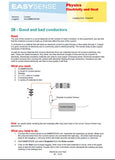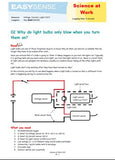# Voltage - Differential 0-10V

datalogging sensors

3161

### This Voltage sensor measures the potential difference across any component in d.c. circuits.

53-sec capacitor - charge & dischargeTeaching applications:

• Introductory electricity
• Ohm’s law
• Simple series and parallel circuits
• Startup of a filament lamp

The 4 mm plugs attach to most school based power supplies and available electricity kits. This sensor can be used anywhere within a circuit. This voltage sensor is designed to show only positive readings.

Sensor Ranges

Range Name Value Resolution Accuracy
Voltage Differential 0-10V 10mV

Other Specifications:

 Maximum Voltage ±27V Impedance 1Meg ohm

### Contents/Details:

0 to 10 V (Resolution 10 mV)
Maximum Voltage ±27 V, Impedance 1 Meg ohmGood and bad conductors (Physics (14-18) : Electricity & Heat eBook)
This activity is a standard practical comparing current flow with different materials in a circuit. Strips of different conductors and insulators are provided for the pupils. Conductors are anything that let a current flow.

Connecting resistors (Physics (14-18) : Electricity & Heat eBook)
Shows the current in circuit with different resistor values and combinations. Leads to resistor calculations.

Electrical characteristics (LED, resistor and filament lamp) (Physics (14-18) : Electricity & Heat eBook)
Create voltage current curves for LED, resistor and filament lamp. This worksheet assumes all three curves in one teaching session. 35a and 35b restrict to fewer devices per session.

Electrical characteristics (resistor and filament lamp) (Physics (14-18) : Electricity & Heat eBook)
Specific worksheet to produce voltage current curves for a resistor and filament lamp (ohmic and non ohmic resistance)

Electrical characteristics (Led, diode) (Physics (14-18) : Electricity & Heat eBook)
Restricted instructions for voltage current curves for diodes.

Time constant of a capacitor (Physics (14-18) : Electricity & Heat eBook)
A good example of data logging enhancing work. collect voltage (and current data, if required) to study time constants and exponential decay. A very good practical.Why do bulbs blow (Science At Work (11-16) eBook)
This activity uses this familiar event to introduce the idea of power surges and to calculate resistance changes. It is a practical introduction to the relationship between voltage and current and the problem of correctly fusing devices in a home for safety.

HK\$ 484.00

3161